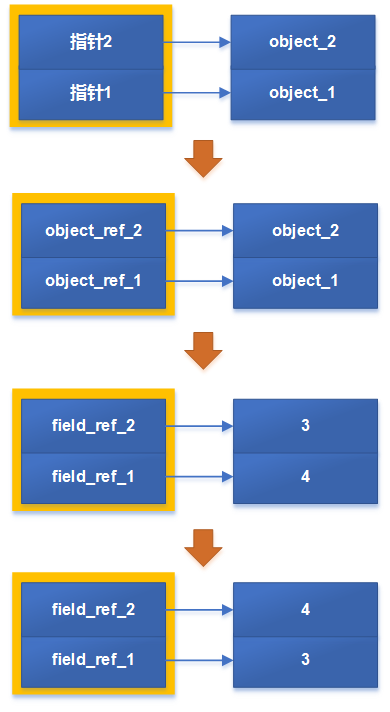# 你会swap吗，按值传递还是按引用？

## 问题##

1、Java到底是按值传递(Call by Value)，还是按引用传递(Call by Reference)？
2、如下面的代码，为什么不能进行交换？

public CallBy swap2(CallBy a,CallBy b) {
CallBy t = a;
a = b;
b = t;
return b;
}


3、如下面的代码，为什么能够交换成功？

public int swap2(CallBy a,CallBy b) {
int t = a.value;
a.value = b.value;
b.value  = t;
return t;
}


## 简单的C++例子##

#include <iostream>

using namespace std;

// 可以交换的例子
void call_by_ref(int &p,int &q) {
int t = p;
p = q;
q = t;
}

// 不能交换的例子
void call_by_val_ptr(int * p,int * q) {
int * t = p;
p = q;
q = t;
}

// 不能交换的例子
void call_by_val(int p,int q){
int t = p ;
p = q;
q = t;
}

int main() {
int a = 3;
int b = 4;

cout << "---------- input ------------" << endl;
cout << "a = " << a << ", b = " << b << endl << endl;

call_by_val(a,b);
cout << "---------- call_by_val ------------" << endl;
cout << "a = " << a << ", b = " << b << endl << endl;

call_by_val_ptr(&a,&b);
cout << "---------- call_by_val_ptr ------------" << endl;
cout << "a = " << a << ", b = " << b << endl << endl;

call_by_ref(a,b);
cout << "---------- call_by_ref ------------" << endl;
cout << "a = " << a << ", b = " << b << endl;
}


### 1、call_by_ref###void call_by_ref(int &p,int &q) {
push   %rbp
mov    %rsp,%rbp
mov    %rdi,-0x18(%rbp)
mov    %rsi,-0x20(%rbp)

//int t = p;
mov    -0x18(%rbp),%rax
//关键点：rax中存放的是变量的实际地址，将地址处存放的值取出放到eax中
mov    (%rax),%eax
mov    %eax,-0x4(%rbp)

//p = q;
mov    -0x20(%rbp),%rax
//关键点：rax中存放的是变量的实际地址，将地址处存放的值取出放到edx
mov    (%rax),%edx
mov    -0x18(%rbp),%rax
mov    %edx,(%rax)

//q = t;
mov    -0x20(%rbp),%rax
mov    -0x4(%rbp),%edx
//关键点：rax存放的也是实际地址，同上.
mov    %edx,(%rax)
}


1、要有实际地址.
2、要有将值存入实际地址的动作.

// c代码
call_by_val_ptr(&a,&b);

// 对应的汇编代码

lea    -0x18(%rbp),%rdx
lea    -0x14(%rbp),%rax
mov    %rdx,%rsi
mov    %rax,%rdi
callq  4008c0 <_Z11call_by_refRiS_>



### 2、call_by_val###call_by_val的反汇编代码如下：

void call_by_val(int p,int q){
push   %rbp
mov    %rsp,%rbp
mov    %edi,-0x14(%rbp)
mov    %esi,-0x18(%rbp)

//int t = p ;
mov    -0x14(%rbp),%eax
mov    %eax,-0x4(%rbp)

//p = q;
mov    -0x18(%rbp),%eax
mov    %eax,-0x14(%rbp)

//q = t;
mov    -0x4(%rbp),%eax
mov    %eax,-0x18(%rbp)
}


// c++代码
call_by_val(a,b);

// 对应的汇编代码
mov    -0x18(%rbp),%edx
mov    -0x14(%rbp),%eax
mov    %edx,%esi
mov    %eax,%edi
callq  400912 <_Z11call_by_valii>


### 2、call_by_val_ptr###void call_by_val_ptr(int * p,int * q) {
push   %rbp
mov    %rsp,%rbp
mov    %rdi,-0x18(%rbp)
mov    %rsi,-0x20(%rbp)
//int * t = p;
mov    -0x18(%rbp),%rax
mov    %rax,-0x8(%rbp)
//p = q;
mov    -0x20(%rbp),%rax
mov    %rax,-0x18(%rbp)
//q = t;
mov    -0x8(%rbp),%rax
mov    %rax,-0x20(%rbp)
}


// c++代码
call_by_val_ptr(&a,&b);

// 对应的汇编代码
lea    -0x18(%rbp),%rdx
lea    -0x14(%rbp),%rax
mov    %rdx,%rsi
mov    %rax,%rdi
callq  4008ec <_Z15call_by_val_ptrPiS_>


call_by_val_ptr中的交换，从汇编代码就能看出，只是交换了指针指向的地址，而没有通过将值存入这个地址而改变地址中的值

## Java##

Java是按值传递的，但可以达到按引用传递的效果。

### Java按值传递的行为###

public CallBy swap2(CallBy a,CallBy b) {
CallBy t = a;
a = b;
b = t;
return b;
}


public com.haoran.CallBy swap2(com.haoran.CallBy, com.haoran.CallBy);
descriptor: (Lcom/haoran/CallBy;Lcom/haoran/CallBy;)Lcom/haoran/CallBy;
flags: ACC_PUBLIC
Code:
stack=1, locals=4, args_size=3
1: astore_3
3: astore_1
5: astore_2
7: areturn
LineNumberTable:
line 45: 0
line 46: 2
line 47: 4
line 48: 6
LocalVariableTable:
Start  Length  Slot  Name   Signature
0       8     0  this   Lcom/haoran/CallBy;
0       8     1     a   Lcom/haoran/CallBy;
0       8     2     b   Lcom/haoran/CallBy;
2       6     3     t   Lcom/haoran/CallBy;


//t = a
1: astore_3

//a = b
3: astore_1

//b = t
5: astore_2



// a = b bytecode
3: astore_1

/***************************************
****************************************/
mov    -0x10(%r14),%rax\n

//----------------------------------------

movzbl 0x1(%r13),%ebx
inc    %r13
movabs $0x7ffff71ad900,%r10 jmpq *(%r10,%rbx,8)  /*************************************** * astore_1对应的汇编（未优化） ****************************************/ pop %rax mov %rax,-0x8(%r14) //---------------------------------------- movzbl 0x1(%r13),%ebx inc %r13 movabs$0x7ffff71ae100,%r10
jmpq   *(%r10,%rbx,8)


### Java按引用传递的行为###public int swap2(CallBy a,CallBy b) {
int t = a.value;
a.value = b.value;
b.value  = t;
return t;
}


public com.haoran.CallBy swap2(com.haoran.CallBy, com.haoran.CallBy);
descriptor: (Lcom/haoran/CallBy;Lcom/haoran/CallBy;)Lcom/haoran/CallBy;
flags: ACC_PUBLIC
Code:
stack=2, locals=4, args_size=3
1: getfield      #2                  // Field value:I
4: istore_3
7: getfield      #2                  // Field value:I
10: putfield      #2                  // Field value:I
15: putfield      #2                  // Field value:I
19: areturn
LineNumberTable:
line 41: 0
line 42: 5
line 43: 13
line 44: 18
LocalVariableTable:
Start  Length  Slot  Name   Signature
0      20     0  this   Lcom/haoran/CallBy;
0      20     1     a   Lcom/haoran/CallBy;
0      20     2     b   Lcom/haoran/CallBy;
5      15     3     t   I


a.value = b.value;


// itos
{
__ pop(itos);
if (!is_static) pop_and_check_object(obj);
// 关键点：只看这里
__ movl(field, rax);
if (!is_static) {
patch_bytecode(Bytecodes::_fast_iputfield, bc, rbx, true, byte_no);
}
__ jmp(Done);
}


void Assembler::movl(Address dst, Register src) {
InstructionMark im(this);
prefix(dst, src);
emit_int8((unsigned char)0x89);
emit_operand(src, dst);
}


movl对应的汇编代码：

// 对应的关键汇编代码
...
mov    %eax,(%rcx,%rbx,1)
...


## 另一种交换值的方式##

### call_by_WHAT###

void call_by_WHAT(int * p,int * q) {
int t = *p;
*p = *q;
*q = t;
}


int main() {
int a = 3;
int b = 4;

cout << "---------- input ------------" << endl;
cout << "a = " << a << ", b = " << b << endl << endl;

call_by_WHAT(&a,&b);
cout << "---------- call_by_WHAT ------------" << endl;
cout << "a = " << a << ", b = " << b << endl;
}


// 输出

---------- input ------------
a = 3, b = 4

---------- call_by_WHAT ------------
a = 4, b = 3



1、指针p、q或者对象引用objectRef，能够直接指向对象的实际地址。
2、要有一个将值放入实际地址的操作：

// C/C++
*p = *q;
...

// Java
putField -> a.value = b.value
...

// 汇编
mov reg_src , (reg_dst)